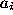Next: Basic Principles Up: Algorithms for Group Actions Previous: Algorithms for Group Actions

# Introduction

The problem of generating graphs has been considered by many authors. We mention only those approaches which are closely related to our work. Mostly the approaches use some kind of orderly generation of which we will present our subset-oriented version below. The basic ideas are from R. Read [R] and I. Faradzhev [F]. An important step forward was made by L.A. Goldberg [Go], who showed that generating graphs by iteratively adding vertices of maximal degree to smaller graphs can achieve a polynomial delay, i.e. a polynomial upper bound for the waiting time, before outputting the first graph and for either outputting the next graph or halting. This was a successful attempt to use structure information in the orderly generation approach. A different strategy had been chosen in the famous DENDRAL project which was an early version of a generator of chemical isomers [LBFL], [GKL]. In that application a 'brutto formula' is given. This details the number of atoms of the different kinds that have to appear in the molecule. The different molecules that correspond to the given brutto formula are called isomers. All isomers that correspond to this input have to be constructed. Since all atoms of the same kind are supposed to have the same valence, we can represent them as vertices of a multigraph regarding the valence as the degree. Thus, in graph theoretical terms, all multigraphs have to be constructed up to isomorphism, where the number of vertices of each degree is prescribed. We callthe degree partition of a graph G, if G hasvertices of degree i for each i. Often in practical applications, some parts of the molecule are known. Then one can replace a subgraph by a vertex of a sometimes high degree. Therefore, though valences of atoms are usually very low, we do consider also vertices of higher degree. Later the vertex has to be expanded to the subgraph. This expansion process is a generator problem of its own and it may again cause isomorphism problems.

We sketch the strategy of the DENDRAL system. The given structure information is analysed to deduce properties of subgraphs which are used as building blocks for the final structures. This process can be explained by considering the construction process in reverse order. Starting from a hypothetical solution, a series of simplification steps are carried through until only small parts remain. So, in a "planning step" the vertices of degree 1 and 2 are removed and cyclic components are formed by removing bridges. From the given brutto formula, the possible number of cyclic components, the possible edge degree series for each cyclic component, and the number of interconnections of these components are deduced. The isomers are then built, up to isomorphism, from a catalogue of cyclic structures. Each construction step requires the solvution of isomorphism problems using some computational group theory. Thus, a structural analysis of the problem makes it possible to break down the problem into smaller pieces which are handled more easily.

The approach presented here makes use of both kinds of ideas. On one hand, the mathematical idea of homomorphism is exploited to use structural information algorithmically. This leads to a recursive construction of (simple) graphs having a given degree partition from regular graphs. Compared to DENDRAL, this allows an unbounded number of simplification steps. On the other hand, orderly generation is used in the remaining homomorphically irreducible cases. The result is a generator which is extremely fast in many situations but which, due to the mathematical overhead, is slow in smaller cases compared to existing generators (such as, for example, B.D. McKay's makeg [McKb] based on the well known isomorphism testing program nauty [McKa], or the present MOLGEN generator [GKLM]). The restriction to simple graphs in this paper is not essential. We only intend to give a mathematical version of the new recursive strategy before adapting it more closely to the needs of computational chemistry.

A complexity analysis is still missing and should evolve out of a search for the best recursion strategy among different variants of the general approach. We intend to look for properties bounding the sizes of the orbit problems that have to be solved in the recursion steps. Generally the use of a series of homomorphic simplifications will lead to a remarkable reduction of the complexity of the task of finding orbit representatives,[L]. In that way, at least in practically important cases, a surprisingly good bound should be possible.